# NCERT Solutions Class 10 Maths Chapter 3 PAIR OF LINEAR EQUATIONS IN TWO VARIABL

Ncert Solutions for class 10 subject Maths Chapter 3 PAIR OF LINEAR EQUATIONS IN TWO VARIABLin pdf Best Free NCERT Solutions for class 1 to 12 in pdf NCERT Solutions, cbse board, Maths, ncert Solutions for Class 10 Maths, class 10 Maths ncert solutions, PAIR OF LINEAR EQUATIONS IN TWO VARIABL, Class 10, ncert solutions chapter 3 PAIR OF LINEAR EQUATIONS IN TWO VARIABL, class 10 Maths, class 10 Maths ncert solutions, Maths ncert solutions class 10, Ncert Solutions Class 10 Mathematics Chapter 3 PAIR OF LINEAR EQUATIONS IN TWO VARIABL

## Concept Chapter 3 Class 10 Maths PAIR OF LINEAR EQUATIONS IN TWO VARIABL Ncert Solutions

< align="justify" style="color:#0033FF; line-height:30px; font-size:18px;">< style="text-align:justify; font-size:16px; color:#0033FF; line-height:30px;">

Linear equations: Two linear equations in the same two variables are called a pair of linear equations in two variables. The most general form of a pair of linear equations is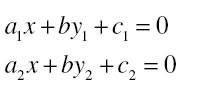Where a1, a2, b1, b2, c1, c2 are real numbers, such that

Method of solving linear equation of two variables
A pair of linear equations in two variables can be represented, and solved, by the:
(i)graphical method (ii) algebraic methodAll in one table for this chapter
If a pair of linear equations are
Then

 S.N. Compare the Ratio Graphic representation Algebraic interpretation Linear equations 1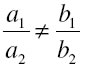Intersection lines at one point Exactly one solution or unique solution Consistent 2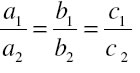Coincident line Infinity solution or many solutions Dependent and consistent 3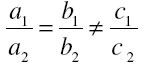Parallel lines No solution Inconsistent

Conclusion from the table

Graphical Method :
The graph of a pair of linear equations in two variables is represented by two lines.
(i) If the lines intersect at a point, then that point gives the unique solution of the two equations. In this case, the pair of equations is consistent.
(ii) If the lines coincide, then there are infinitely many solutions — each point on the line being a solution. In this case, the pair of equations is dependent (consistent).
(iii) If the lines are parallel, then the pair of equations has no solution. In this case, the pair of equations is inconsistent.

Algebraic Methods : We have discussed the following methods for finding the solution(s) of a pair of linear equations :
(i) Substitution Method
(ii) Elimination Method
(iii) Cross-multiplication Method
Formula of cross multiplication is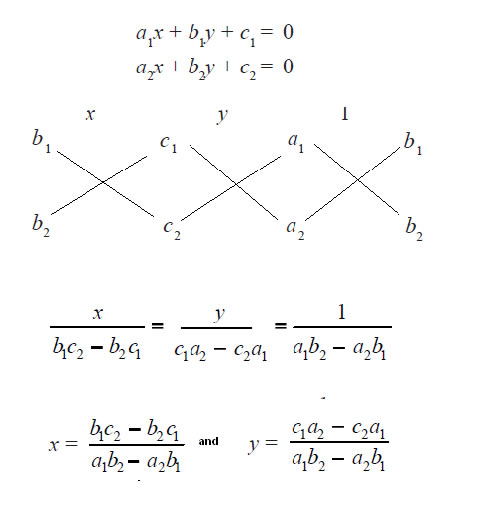## Exercise 3.1 Chapter 3 Class 10 Maths PAIR OF LINEAR EQUATIONS IN TWO VARIABL Ncert Solutions

Question 1. Aftab tells his daughter, “Seven years ago, I was seven times as old as you were then .Also, three years from now, I shall be three times as old as you will be.” (Isn’t this interesting?) Represent this situation algebraically and graphically.

Solution:

Let
age of Aftab is represented by x
age of daughter is represented by y
Then Seven years ago,
age of Aftab = x -7
age of daughter = y-7
given that I was seven times as old as you were so we get
x - 7 = 7 ( y – 7 )
x – 7 = 7 y – 49
x- 7 y = - 49 + 7
x – 7 y = - 42 …(1)
x = 7 y – 42
plug y = 5,6 and 7 we get
x = 7 * 5 - 42 = 35 - 42 = - 7
x = 7 * 6 - 42 = 42 – 42 =0
x = 7 * 7 – 42 = 49 – 42 = 7

 X -7 0 7 Y 5 6 7

three years from now ,
Age of Aftab = x +3
Age of daughter = y+3
Given that I shall be three times as old as you will be so we get
x + 3 = 3 (y + 3)
x + 3 = 3 y + 9
x -3 y = 9- 3
x -3 y = 6 …(2)
x = 3 y + 6
plug y = -2,-1and 0 we get
x = 3 * - 2 + 6 = -6+6 =0
x = 3 * - 1 + 6 = -3+ 6 = 3
x = 3 * 0 + 6 = 0 + 6 = 6

 X 0 3 6 y -2 -1 0

Algebraic representation
From equation (1) and (2)
x – 7 y = – 42 …(1)
x - 3 y = 6 …(2)
Graphical representation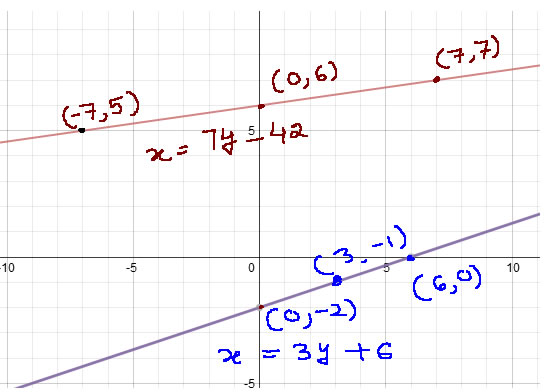Question 1. The coach of a cricket team buys 3 bats and 6 balls for Rs 3900. Later, she buys another bat and 2 more balls of the same kind for Rs 1300. Represent this situation algebraically and geometrically.

Solution: Let cost of one bat= Rs x
Cost of one ball= Rs y
3 bats and 6 balls for Rs 3900 So that
3 x + 6y = 3900… (1)
Divide complete equation by 3 we get
X+ 2y = 1300
Subtract 2y both side we get
X = 1300 – 2y

Plug y = - 1300, 0 and 1300 we get
X = 1300 – 2 ( - 1300 ) = 1300 + 2600 = 3900
X= 1300 -2(0) = 1300 -0 = 1300
X= 1300 – 2(1300) = 1300 – 2600 = - 1300

 X 3900 1300 -1300 y -1300 0 1300

Given that she buys another bat and 2 more balls of the same kind for Rs 1300
So we get
x + 2 y = 1300 … (2)
Subtract 2y both side we get
X = 1300 – 2y
Plug y = - 1300, 0 and 1300 we get
X = 1300 – 2 ( - 1300 ) = 1300 + 2600 = 3900
X = 1300 – 2 ( 0 ) = 1300 - 0 = 1300
X = 1300 – 2(1300) = 1300 – 2600 = -1300

 X 3900 1300 -1300 y -1300 0 1300

Algebraic representation
3 x + 6 y = 3900… (1)
x + 2 y = 1300 … (2)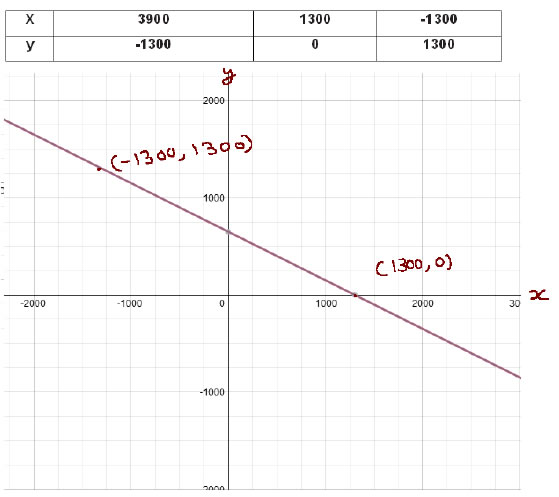Question 3. The cost of 2 kg of apples and 1kg of grapes on a day was found to be Rs 160. After a month, the cost of 4 kg of apples and 2 kg of grapes is Rs 300. Represent the situation algebraically and geometrically.

Solution: Let cost each kg of apples = Rs x
Cost of each kg of grapes = Rs y
Given that The cost of 2 kg of apples and 1kg of grapes on a day was found to be Rs 160
So that
2 x + y = 160 … (1)
2x = 160 - y
x = (160 – y)/2
Let y = 0 , 80 and 160 we get
X = (160 – ( 0 ))/2 = 80
X = (160- 80 )/2 = 40
X = (160 – 2* 80 )/2 = 0

 X 80 40 0 y 0 80 160

Given that the cost of 4 kg of apples and 2 kg of grapes is Rs 300
so we get
4 x + 2 y = 300 … (2)
Divide by 2 we get
2 x + y = 150
Subtract 2x both side we get
Y = 150 – 2 x
Plug x = 0 , 50 , 100 we get
Y = 150 – 2*0 = 150
Y = 150 – 2* 50 = 50
Y = 150 – 2 * (100 ) = - 50

 X 0 50 100 Y 150 50 -50

Algebraic representation
2 x + y = 160 … (1)
4 x + 2 y = 300 … (2)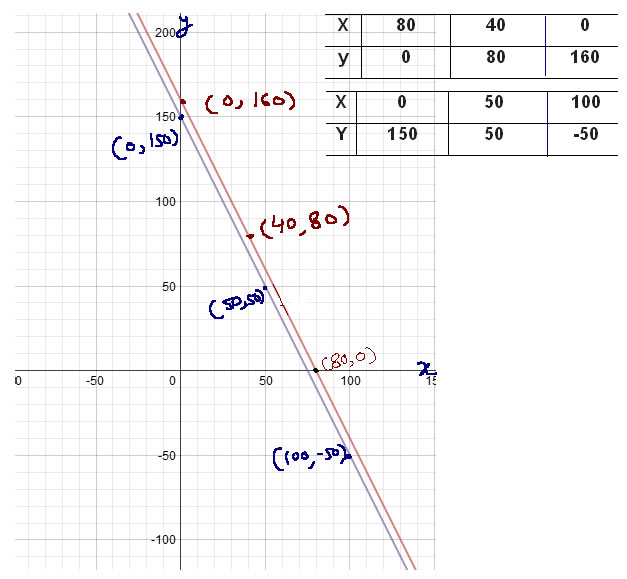## Exercise 3.2 Chapter 3 Class 10 Maths PAIR OF LINEAR EQUATIONS IN TWO VARIABL Ncert Solutions

Question 1. Form the pair of linear equations in the following problems, and find their solutions graphically.
(i) 10 students of Class X took part in a Mathematics quiz. If the number of girls is 4 more than the number of boys, find the number of boys and girls who took part in the quiz.
Solution:
Let number of boys = x
Number of girls = y
Given that total number of student is 10 so that
x + y = 10
Subtract y both side we get
x = 10 – y
Plug y = 0 , 5, 10 we get
X = 10 – 0 = 10
X = 10 – 5 = 5
X = 10 – 10 = 0

 X 10 5 0 Y 0 5 10

Given that If the number of girls is 4 more than the number of boys
So that
y = x + 4
Plug x = -4, 0, 4, and we get
Y = - 4 + 4 = 0
Y = 0 + 4 = 4
Y = 4 + 4 = 8

 X -4 0 4 y 0 4 8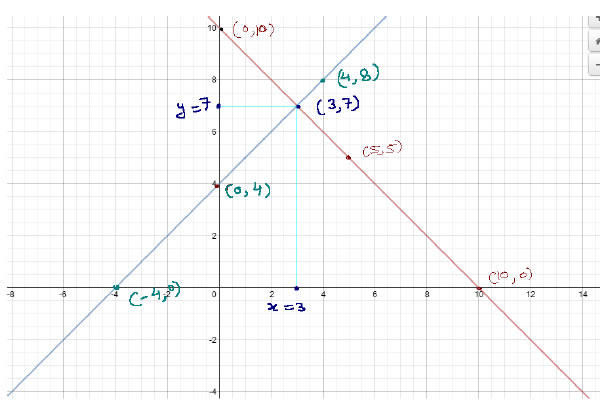So number of boys = 3 and number of girls = 7

Question 1(ii). 5 pencils and 7 pens together cost Rs 50, whereas 7 pencils and 5 pens together cost Rs 46. Find the cost of one pencil and that of one pen
Solution:
Let cost of pencil = Rs x
Cost of pens= Rs y

5 pencils and 7 pens together cost Rs 50,
So we get
5x + 7y = 50
Subtract 7y both side we get
5x = 50 – 7 y
Divide by 5 we get
X = 10 - 7 y /5
Plug value of y which is factor of 5 to get whole number so plug y = 5 , 10 and 15 we get
X = 10 – 7 * 5 / 5 = 10 – 7 = 3
X = 10 – 7 * 10 / 5 = 10 – 14 = - 4
X = 10 – 7 * 15 / 5 = 10 – 21 = - 11

 X 3 -4 -11 y 5 10 15

Given that 7 pencils and 5 pens together cost Rs 46
7 x + 5 y = 46
Subtract 7x both side we get
5y = 46 – 7x
Divide by 5 we get
Y = 46 / 5 - 7 x / 5
Y = 9.2 – 1.4 x
Plug x = 0 , 2 and 4 we get
Y = 9.2 – 1.4 * 0 = 9.2 – 0 = 9.2
Y = 9.2 – 1.4 (2) = 9.2 – 2.8 =6.4
Y = 9.2 – 1.4 (4) = 9.2 – 5.6 = 3.6

 X 0 2 4 Y 9.2 6.4 3.6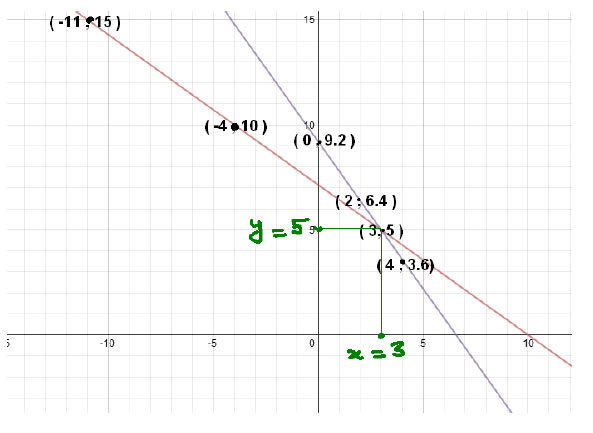Answer cost of 1 pencil = Rs 3Cost of 1 pen = Rs 5

Question 2. On comparing the ratios a1/a2 , b1/b2 and c1/c2, find out whether the lines representing the following pairs of linear equations intersect at a point, are parallel or coincident:
Use this table for the solution

 S.N. Compare the Ratio Graphic representation Algebraic interpretation Linear equations 1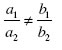Intersection lines at one point Exactly one solution or unique solution Consistent 2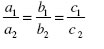Coincident line Infinity solution or many solutions Dependent and consistent 3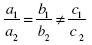Parallel lines No solution Inconsistent

Solution: (i)

5x – 4y + 8 = 0

7x + 6y – 9 = 0
Compare the equation with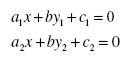We get
a1 = 5,b1 = -4, and c1 = 8
a2 =7 b2 =6 and c2 = -9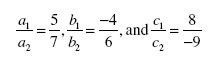Hence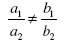So both are Intersecting lines at one point
(ii)9x + 3y + 12 = 0
18x + 6y + 24 = 0
Compare the equation withWe get
a1 = 9, b1 = 3, and c1 = 12
a2 =18 b2 =6, and c2 = 24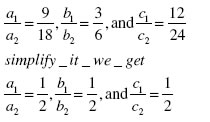HenceSo both lines are coincident

(iii)6x – 3y + 10 = 0
2x – y + 9 = 0
Compare the equation withWe get
a1 = 6,b1 =- 3, and c1 = 10
a2 =2 b2 =-1, and c2 = 9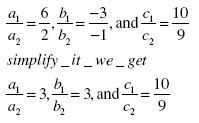HenceSo both lines are parallel

Question 3. On comparing the ratios a1/a2 , b1/b2 and c1/c2 find out whether the following pair of linear equations are consistent, or inconsistent.
(i) 3x + 2y = 5 ; 2x – 3y = 7
(ii) 2x – 3y = 8 ; 4x – 6y = 9
(iii) 3/2x + 5/3 y = 7 ; 9x – 10y = 14
(iv) 5x – 3y = 11 ; – 10x + 6y = –22
(v)4/3x + 2y =8 ; 2x + 3y = 12
(i) 3x + 2y = 5 ; 2x – 3y = 7
Solution:
Convert the equation in form of a1x + b1y + c1 = 0 and a2x+ b2y + c2 = 0
We get
3x + 2y - 5 =0 and 2x – 3y – 7 =0
Compare the equation withWe get
a1 = 3,b1 = 2, and c1 = -5
a2 =2 b2 =-3 and c2 = -7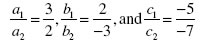We getHence both lines are Consistent
(ii) 2x – 3y = 8 ; 4x – 6y = 9
Convert the equation in form of a1x + b1y + c1 = 0 and a2x+ b2y + c2 = 0
We get
2 x – 3 y - 8 = 0 and 4x – 6 y – 9 =0
Compare the equation withWe get
a1 = 2,b1 = -3, and c1 = - 8
a2 = 4 b2 = - 6 and c2 = - 9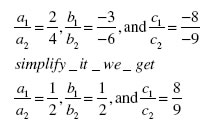So we getSo both lines are Inconsistent
(iii) 3/2x + 5/3 y = 7 ; 9x – 10y = 14
Convert the equation in form ofa1x + b1y + c1 = 0 and a2x+ b2y + c2 = 0
We get
3/2 x + 5/3 y - 7 = 0 and 9x – 10 y - 14 =0
Compare the equation withWe get
a1 = 3/2,b1 = 5/3, and c1 = - 7
a2 = 9 b2 = - 10 and c2 = - 14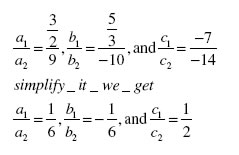So we get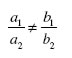So both lines are Consistent
(iv) 5x – 3y = 11 ; – 10x + 6y = –22
Convert the equation in form ofa1x + b1y + c1 = 0 and a2x+ b2y + c2 = 0
We get
5 x -3 y - 11 = 0 and -10 x + 6 y + 22 =0
Compare the equation withWe get
a1 = 5 b1 = - 3, and c1 = - 11
a2 = -10 b2 = 6 and c2 = 22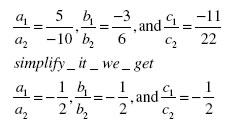So we getHence
So both lines are dependent and consistent
(v)4/3x + 2y =8 ; 2x + 3y = 12
Convert the equation in form of a1x + b1y + c1 = 0 and a2x+ b2y + c2 = 0
We get
4/3 x + 2 y - 8 = 0 and 2x + 3 y - 12 =0
Compare the equation withWe get
a1 = 4/3,b1 = 2, and c1 = -8
a2 = 2 b2 = 3 and c2 = - 12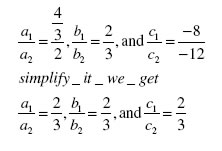So we getSo both lines are Dependent and consistent

Question 4. Which of the following pairs of linear equations are consistent/inconsistent? If consistent, obtain the solution graphically:

(i) x + y = 5, 2x + 2y = 10

Convert the equation in form ofa1x + b1y + c1 = 0 and a2x+ b2y + c2 = 0
We get
x + y -5 = 0, 2 x + 2 y -10 = 0
Compare the equation withWe get
a1 = 1,b1 = 1, and c1 = -5
a2 = 2 b2 = 2 and c2 = - 10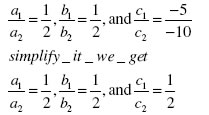So we getDependent and consistent so it will have many solutions .

(ii) x – y = 8, 3x – 3y = 16

Convert the equation in form of
a1x + b1y + c1 = 0 and a2x+ b2y + c2 = 0
We get
x – y -8 = , 3x – 3y -16 = 0
Compare the equation withWe get
a1 = 1, b1 = - 1, and c1 = - 8
a2 = 3 b2 = - 3 and c2 = - 16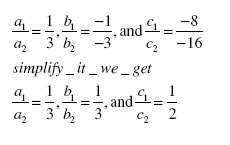So we getSo both lines are Inconsistent
(iii) 2x + y – 6 = 0, 4x – 2y – 4 = 0
Compare the equation withWe get
a1 = 2,b1 = 1, and c1 = - 6
a2 = 4 b2 = - 2 and c2 = - 4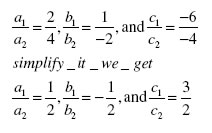So we getSo both lines are consistent

Solve the equations graphically
2x + y – 6 = 0,
Subtract 2x and add 6 both side we get
y = 6 – 2x
plug x = 1,2 and 3 we get
y = 6 - 2 * 1 = 4
y = 6 - 2 * 2 = 2
y = 6 - 2 * 3 = 0

 X 1 2 3 y 4 2 0

4 x – 2 y – 4 = 0
Divide by 2 we get
2 x – y - 2 = 0
Add y both side we get
2 x – 2 = y
or
y = 2x – 2
plug x = 1.2 and 3 we get
y = 2 * 1 – 2 = 0
y = 2 * 2 – 2 = 2
y = 2 * 3 – 2 = 4

 X 1 2 3 y 0 2 4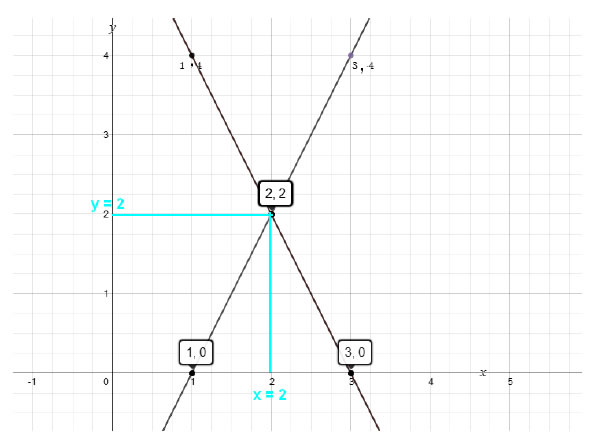Answer x = 2 and y = 2

(iv) 2x – 2y – 2 = 0, 4x – 4y – 5 = 0
Compare the equation withWe get
a1 = 2,b1 = - 2, and c1 = - 2
a2 = 2 b2 = - 2 and c2 = - 5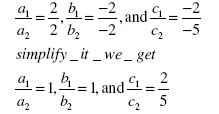So we getSo both lines are Inconsistent

Question 5. Half the perimeter of a rectangular garden, whose length is 4 m more than its width, is 36 m. Find the dimensions of the garden.

Solution: Let length of rectangle = x m
With of the rectangle = y m
Given that whose length is 4 m more than its width
so that
x = 4 + y
plug y = -18 , 0, and 18 we get
x = 4 - 18 = -14
x = 4 +0 = 0
x = 4 + 18 = 22

 X -14 0 22 y -18 0 18

Given that Half of the perimeter of the rectangle is 36 m so
Formula of perimeter of the rectangle = 2(x+y)
Half of perimeter of rectangle = (1/2)*2(x + y) = x + y
So our equation will
x + y = 36
Subtract y both side we get
X = 36 – y
Plug y = 36 ,18 and 0 we get
X = 36 – 36 = 0
X = 36 – 18 = 18
X = 36 – 36 = 0

 X 0 18 36 y 36 18 0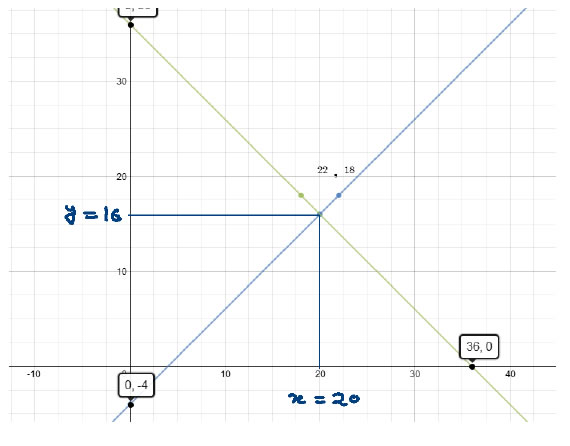Hence length of garden = 20 m Width of garden = 16 m

Question 6. Given the linear equation 2x + 3y – 8 = 0, write another linear equation in two variables such that the geometrical representation of the pair so formed is:
Solution:
(i) intersecting lines
2x + 3y – 8 = 0
The condition for intersecting lines is
So we multiply any value with a1 and not with b1
If we multiply by 2 second such equation will
4x + 3y – 8 = 0

(ii)parallel lines

the condition for parallel lines isSo multiply with a1 , b1 with any number and not multiply with c
if we multiply by 2 then second equation will
4x + 6y – 8 = 0

(iii) coincident lines

the condition for coincident lines areSo multiply by any number with whole equation
If we multiply by 2 we get
4x + 6y – 16 = 0

Question 7. Draw the graphs of the equations x – y + 1 = 0 and 3x + 2y – 12 = 0. Determine the coordinates of the vertices of the triangle formed by these lines and the x-axis, and shade the triangular region.
Solution:

First equation is x – y + 1 = 0
Add y and subtract 1 both side we get
X = y -1
Plug y = 0 ,1 and 2 we get
X = 0 -1 = - 1
X = 1 -1 = 0
X = 2 -1 = 1

 X -1 0 1 Y 0 1 2

3x + 2y – 12 = 0
Subtract 3x and add 12 both side we get
2y = 12 – 3x
Divide by 2 we get
Y = 6- 1.5 x
Plug x = 0 ,2, and 4 we get
Y = 6- 1.5 *0 = 6
Y = 6- 1.5 *2 = 3
Y = 6- 1.5 *4 = 0

 X 0 2 4 Y 6 3 0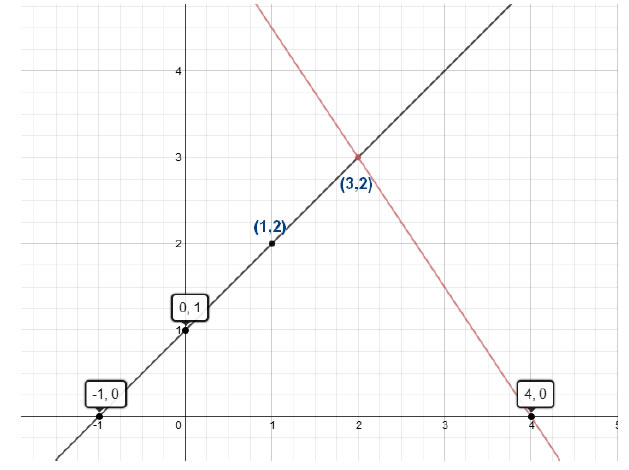Both lines are intersects at x = 3 and y = 2
Hence answer is x = 3 and y = 2

## Exercise 3.3 Chapter 3 Class 10 Maths PAIR OF LINEAR EQUATIONS IN TWO VARIABL Ncert Solutions

Question 1. Solve the following pair of linear equations by the substitution method.
(i) x + y = 14 ; x – y = 4 (ii) s – t = 3 ; s/3 + t/2 = 6
(iii) 3x – y = 3 ; 9x – 3y = 9 (iv) 0.2x + 0.3y = 1.3 ; 0.4x + 0.5y = 2.3
(v)√2 x+ √3y = 0 ; √3 x - √8y = 0 (vi)3/2 x - 5/3y = -2 ; x/3 + y/2 = 13/6

Solution: (i) x + y = 14 ; x – y = 4

solve first equation
x + y = 14
x = 14 - y …………..(1)
plug this value in equation second we get
x – y = 4
14 – y - y = 4
Add 14 both side we get
- 2 y = 4 - 14
- 2 y = - 10
Y = -10/-2
Y = 5
Plug y = 5 in equation first we get
X = 14 – y
X = 14 – 5
X = 9
Answer x = 9 , y = 5

(ii)s – t = 3 ; s/3 + t/2 = 6

Solve first equation
s – t = 3
s = 3+t …………(1)
Plug this value in equation second we get
s/3 + t/2 = 6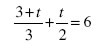Multiply by 6 to remove all denominator we get
6 + 2t + 3t = 36
5t = 36 – 6
5t = 30
Divide by 2 we get
t = 30/5
t =6
Plug the value in equation first we get
s = 3 +6
s = 9
Answer s = 9 , t = 6

(iii)3x – y = 3 ; 9x – 3y = 9

solve first equation
3x – y = 3
3x =3 +y
x = (3 + y)/3 …………..(1)
Plug this value in equation second we get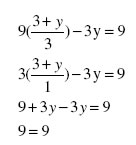9 is always equal to 9
So it is a true condition and in such condition
Equations will have many or infinity solutions

(iv) 0.2x + 0.3y = 1.3 …..(1) ;

0.4x + 0.5y = 2.3 ...(2)
0.2x + 0.3y = 1.3
Solve first equation we get
0.2x = 1.3 – 0.3y
Divide by 0.2 we get
X = 1.3/0.2 - 0.3/0.2
X = 6.5 – 1.5 y …(3)
Plug the value in equation second we get
0.4x + 0.5y = 2.3
(6.5 – 1.5y)*0.4x + 0.5y = 2.3
2.6 – 0.6y + 0.5y = 2.3
-0.1 y = 2.3 – 2.6
Y = - 0.3/-0.1
Y = 3
Plug this value in equation 3 we get
X = 6.5 – 1.5 y
X = 6.5 – 1.5(3)
X =6.5 - 4.5
X =2
Answer x = 2, y = 3

(v)√2 x+ √3y = 0 ...(1)

√3 x - √8y = 0 ... (2)
√2 x+ √3y = 0
Subtract √3y both side we get
√2 x = -√3y
Divide by √2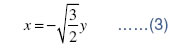Plug the value of x in equation first we get
√3 x - √8y = 0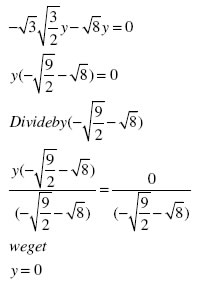Plug this value in equation (3) we get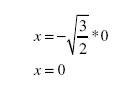Our answer x = 0 and y = 0

(vi) 3/2 x - 5/3y = -2 ……(1)

x/3 + y/2 = 13/6 .......(2)
Solve first equation
3/2 x - 5/3y = -2
Multiply by 6 to remove all denominator we get
9 x – 10 y = -12
9x = -12 + 10y
Divide by 9 we get
x = (-12 + 10 y)/9 …..(3)
plug this value in equation (2) we get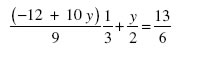Multiply by LCM of denominator = 27* 2 = 54 we get
2(-12 +10 y ) + 27 y= 117
-24 + 20 y + 27 y = 117
47 y = 117 + 24
Y = 141/47 = 3
Plug this value in equation (3) we get
x = (-12 + 10*3)/9
x=18/9
x = 2
Answer x = 2 and y = 3

Question 2. Solve 2x + 3y = 11 and 2x – 4y = – 24 and hence find the value of ‘m’ for which y =mx + 3.
Solution:
2x + 3y = 11 ... (1)
Subtract 3y both side we get
2x = 11 – 3y … (3)
Plug this value in equation second we get
2x – 4y = – 24 … (2)
11- 3y – 4y = - 24

7 y = - 24 – 11

-7y = - 35
y = - 35 / - 7
y = 5
Plug this value in equation (3) we get
2x = 11 – 3* 5
2x = 11- 15
2x = - 4
Divide by 2 we get
x = - 2
Plug the value of x and y in equation
Y =mx + 3.
5 = -2m +3
2 m = 3 – 5
M = - 2 / 2
M = - 1
Answer x = - 2 , 5 and m = -1

Question 3. Form the pair of linear equations for the following problems and find their solution by substitution method.
(i)The difference between two numbers is 26 and one number is three times the other. Find them.
(ii) The larger of two supplementary angles exceeds the smaller by 18 degrees. Find them.
(iii) The coach of a cricket team buys 7 bats and 6 balls for Rs 3800. Later, she buys 3 bats and 5 balls for Rs 1750. Find the cost of each bat and each ball.
(iv) The taxi charges in a city consist of a fixed charge together with the charge for the distance covered. For a distance of 10 km, the charge paid is Rs 105 and for a journey of 15 km, the charge paid is Rs 155. What are the fixed charges and the charge per km? How much does a person have to pay for travelling a distance of 25 km?
(v) A fraction becomes 9/11, if 2 is added to both the numerator and the denominator. If, 3 is added to both the numerator and the denominator it becomes 5/6 . Find the fraction.
(vi) Five years hence, the age of Jacob will be three times that of his son. Five years ago, Jacob’s age was seven times that of his son. What are their present ages?

(i)The difference between two numbers is 26 and one number is three times the other. Find them.

Let larger number = x
Smaller number = y
The difference between two numbers is 26
x – y = 26
x = 26 + y
Given that one number is three times the other
So x = 3y
plug the value of x we get

26 + y = 3y
-2y = - 26
y = 13
So value of x = 3y
Plug value of y we get
X = 3* 13 = 39
Answer smaller number = 13 bigger number is 39
(ii) The larger of two supplementary angles exceeds the smaller by 18 degrees. Find them.
Let first angle = x
And second number = y

x + y = 180
x = 180 - y ... (1)

x – y = 18
Plug the value of x we get

180– y – y = 18
2 y = -162
y = -162/-2
y = 81
Plug the value back in equation first we get
X = 180 – 81 = 99
Hence angles are 81 and 99

(iii) The coach of a cricket team buys 7 bats and 6 balls for Rs 3800. Later, she buys 3 bats and 5 balls for Rs 1750. Find the cost of each bat and each ball.
Let cost of each bat = Rs x
Cost of each ball = Rs y
Given that coach of a cricket team buys 7 bats and 6 balls for Rs 3800.
So that 7x + 6y = 3800
6y = 3800 – 7x
Divide by 6 we get
y = (3800 – 7x) /6 … (1)
Given that she buys 3 bats and 5 balls for Rs 1750.so that
3x + 5y = 1750
Plug the value of y
3x + 5 ((3800 – 7x) /6) = 1750
Multiply by 6 we get
18 x + 19000 – 35 x = 10500
-17x =10500 - 19000
-17x = -8500
x = - 8500 / - 17
x = 500
Plug this value in equation first we get
Y = ( 3800 – 7 * 500) / 6
Y = 300/6
Y = 50
Hence cost of each bat = Rs 500 and cost of each balls is Rs 50

(iii) The taxi charges in a city consist of a fixed charge together with the charge for the distance covered. For a distance of 10 km, the charge paid is Rs 105 and for a journey of 15 km, the charge paid is Rs 155. What are the fixed charges and the charge per km? How much does a person have to pay for travelling a distance of 25 km?

Let the fixed charge for taxi = Rs x
And variable cost per Km = Rs y
Total cost = fixed charge + variable charge
Given that for a distance of 10 km, the charge paid is Rs 105
x + 10 y = 105 … (1)
x = 105 – 10 y
Given that for a journey of 15 km, the charge paid is Rs 155
x + 15 y = 155
Plug the value of x we get
105 – 10 y + 15 y = 155
5y = 155 – 105
5y = 50
Divide by 5 we get
y = 50 / 5 = 10
Plug this value in equation (1) we get
x = 105 – 10 * 10
x = 5
People have to pay for travelling a distance of 25 km
= X + 25 y
= 5 + 25*10
= 5 + 250
=255
Answer pay for 25 Km is Rs 255
(v) A fraction becomes 9/11, if 2 is added to both the numerator and the denominator. If, 3 is added to both the numerator and the denominator it becomes 5/6 . Find the fraction.
Let Numerator = x
Denominator = y
Fraction will = x/ y
A fraction becomes 9/11, if 2 is added to both the numerator and the denominator
(x+2) = 9
y+211

Cross multiply we get
11 x + 22 = 9y + 18
Subtract 22 both side we get
11 x = 9 y – 4
Divide by 11 we get
x = 9 y – 4
11 … (1)
Given that 3 is added to both the numerator and the denominator it becomes 5/ 6.
If, 3 is added to both the numerator and the denominator it becomes 5/6
(x+3) = 5 … (2)
y + 3 6

Cross multiply we get
6x + 18 = 5y + 15
Subtract the value of x now we get
6(9 y – 4 ) + 18 = 5y + 15
11
Subtract 18 both side we get
6(9 y – 4 ) = 5y - 3
11

54– 24 = 55 y - 33

-y = -9
y = 9
Plug this value of y in equation (1) we get
x = 9 y – 4
11 … (1)
x = (81 – 4)/77
x = 77/11
x = 7

Hence our fraction is 7/9

(vi) Five years hence, the age of Jacob will be three times that of his son. Five years ago, Jacob’s age was seven times that of his son. What are their present ages?
Let present age of Jacob = x year
Andpresent Age of his son is = y year
Five years hence,
Age of Jacob will = x + 5 year
Age of his son will = y + 5year
Given that the age of Jacob will be three times that of his son
x + 5 =3(y+5)
Add 5 both side we get
x = 3y + 15 - 5
x = 3y + 10 … (1)
Five years ago,
Age of Jacob will = x - 5 year
Age of his son will = y - 5year
Jacob’s age was seven times that of his son
x – 5 = 7(y -5)
Plug the value of x from equation (1) we get
3y + 10 – 5 = 7 y – 35
3y + 5 = 7y – 35
3y – 7 y = - 35 – 5
-4y = - 40
y = - 40 / - 4
y = 10 year
Plug the value of y in equation first we get
x = 3* 10 + 10
x = 40 years
Answer is Present age of Jacob = 40 years Present age of his son = 10 years

## Exercise 3.4 Chapter 3 Class 10 Maths PAIR OF LINEAR EQUATIONS IN TWO VARIABL Ncert Solutions

Question 1. Solve the following pair of linear equations by the elimination method and the substitution method:
x + y =5 and 2x –3y = 4
3x + 4y = 10 and 2x – 2y = 2
3x – 5y – 4 = 0 and 9x = 2y + 7
x/2 + 2y /3 = - 1 and x – y/3 = 3

Solution: By elimination method
x + y =5 ……….(1)
2x –3y = 4 ………(2)
Multiplying equation (1) by 2, we obtain
2x + 2y = 10 ………..(3)
2x –3y = 4 ………(2)
Subtracting equation (2) from equation (3), we obtain
5y = 6
y = 6/5
Substituting the value in equation (1), we obtain
x = 5 - (6/5) = 19/5
So our answer is x = 19/5 and y = 6/5

By substitution method
x + y =5 ……….(1)
subtract y both side we get
x = 5 - y ……..,(4)
plug the value of x in equation second we get
2(5 – y ) – 3y = 4
-5y = - 6
y = -6/-5 = 6/5
Plug the value of y in equation 4 we get
x = 5 – 6/5
x = 19/5
So our answer is x = 19/5 and y = 6/5 again

3x + 4y = 10 and 2x – 2y = 2
By elimination method

3x + 4y = 10 ………(1)
2x – 2y = 2 ……….(2)
Multiplying equation (2) by 2, we obtain
4 x – 4 y = 4 ………..(3)
3x + 4y = 10 ………(1)
Adding equation (1) and (3), we obtain
7x + 0 = 14
Divide by 7 both side we get
X = 14/7 = 2
Substituting in equation (1), we obtain
3x + 4y = 10
3(2) + 4 y = 10
6 + 4 y = 10
4y = 10 – 6
4y = 4
y = 4/4 = 1

Hence answer is x = 2, y = 1

By substitution method
3x + 4y = 10 ………(1)
Subtract 3x both side we get
4 y = 10 – 3x

y = (10 - 3x )/4
Plug this value in equation second we get
2x – 2y = 2 ……….(2)
2x – 2(10 - 3x )/4) = 2
Multiply by 4 we get
8x - 2(10 – 3x) = 8
8x - 20 + 6 x = 8
14 x = 28
X = 28/14 = 2
y = (10 - 3x )/4
y = 4/4 = 1
3x – 5y – 4 = 0 and 9x = 2y + 7
By elimination method

3x – 5y – 4 = 0
3x – 5y = 4 ………….(1)
9x = 2y + 7
9x – 2y = 7 ……….(2)
Multiplying equation (1) by 3, we obtain
9 x – 15 y = 11 ……(3)
9x – 2y = 7 ...….(2)

Subtracting equation (2) from equation (3), we obtain
-13 y = 5
y = - 5/13
Substituting in equation (1), we obtain
3x – 5y = 4 ………….(1)
3x - 5(-5/13) = 4
Multiply by 13 we get
39 x + 25 = 52
39 x = 27
X =27/39 = 9 /13
Hence our answer Is x = 9/13 and y = - 5/13
By substitution method
3x – 5y = 4 ………….(1)
Add 5y both side we get
3x = 4 + 5y
Divide by 3 we get
X = (4 + 5y )/3 ……..(4)
Plug this value in equation second we get
9x – 2y = 7 ...….(2)
9 ((4 + 5y )/3) – 2y = 7
Solve it we get
3(4 + 5y ) – 2y = 7
12 + 15 y – 2y = 7
13 y = - 5
y = -5/13
Plug this value back in equation 4 we get
X = (4 + 5y )/3
X = (4 +5* (-5/13))/ 3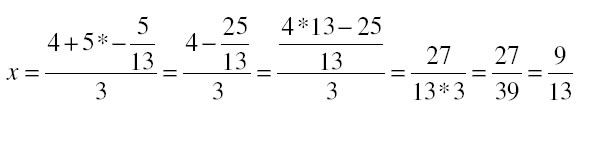Hence we get x = 9/13 and y = - 5/13 again

x/2 + 2y /3 = - 1 and x – y/3 = 3
(iv) By elimination method
x/2 + 2y /3 = - 1 ………..(1)
x – y/3 = 3 ………..(2)
Multiplying equation (1) by 2, we obtain
x + 4y/3 = - 2 ………(3)
x – y/3 = 3 ………..(2)

Subtracting equation (2) from equation (3), we obtain
5y /3 = -5
Divide by 5 and multiply by 3 we get
y = -15/5
y = - 3

Substituting in equation (2), we obtain
x – y/3 = 3 ………..(2)
x – (-3)/3 = 3
x + 1 = 3
x = 2
Hence our answer is x = 2 and y = −3
By substitution method
x – y/3 = 3 ………..(2)
Add y/3 both side we get
x= 3 + y/3 ……(4)
Plug this value in equation (1) we get
x/2 + 2y /3 = - 1 ………..(1)
(3+ y/3)/2 + 2y /3 = -1
3/2 + y /6 + 2y/3 = - 1
Multiply by 6 we get
9 + y + 4y = - 6
5y = -15
y = - 3
Hence our answer is x = 2 and y = −3

Question 2. Form the pair of linear equations in the following problems, and find their solutions (if they exist) by the elimination method:
(i) If we add 1tothe numerator and subtract 1fromthe denominator, a fractionreduces to 1. It becomes1/2 if we only add 1 to the denominator.What is the raction?
(ii) Five years ago, Nuri was thrice as old as Sonu. Ten years later, Nuri will be twice as old as Sonu. How old are Nuri and Sonu?
(iii) The sum of the digits of a two-digit number is 9. Also, nine times this number is twice the number obtained by reversing the order of the digits. Find the number.
(iv) Meena went to a bank to withdraw Rs 2000. She asked the cashier to give her Rs 50 and Rs 100 notes only. Meena got 25 notes in all. Find how many notes of Rs 50 and Rs 100 she received.
A lending library has a fixed charge for the first three days and an additional charge for each day thereafter. Saritha paid Rs 27 for a book kept for seven days, while Susy paid Rs 21 for the book she kept for five days. Find the fixed charge and the charge for each extra day.
(i) If we add 1tothe numerator and subtract 1fromthe denominator, a fraction reduces to 1. It becomes1/2 if we only add 1 to the denominator. What is the fraction?
Let numerator = x
And denominator = y
Fraction = x/y
If we add 1tothe numerator and subtract 1fromthe denominator, a fractionreduces to 1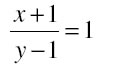Croos multiply we get
x + 1 = y – 1
x- y = -2 ……..(1)
It becomes1/2 if we only add 1 to the denominator.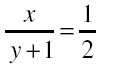Croos multiply we get
2x = y + 1
2x- y = 1 ……..(2)
x- y = -2 ……..(1)
Subtracting equation (1) from equation (2) we get

x = 3

Substituting this value in equation (1) we get
3– y = - 2
- y = - 5
y = 5
Hence our fraction is 3/5
(ii) Fiveyears ago,Nuri was thrice as old as Sonu. Ten years later,Nuri will be twice as old as Sonu. How old are Nuri and Sonu?

Let the present age of Nuri = x year
And present age of Sonu = y year
Five years ago
Age of Nuri = x – 5 years
Age of Sony = y – 5 years
Nuri was thrice as old as Sonu
x- 5 = 3(y – 5)
x – 5 = 35 – 15
x – 3y = -15 + 5
x – 3 y = - 10 ………..(1)
Ten years later,
Age of Nuri = x + 10
Age of Sonu = y + 10
Nuri will be twice as old as Sonu.
x+ 10 = 2(y+10)
x + 10 = 2y + 20
x – 2y = 10 ………..(2)
x – 3 y = - 10 ………..(1)
Subtracting equation (1) from equation (2) we get
y = 20
Plug this value in equation first we get
x - 3* 20 = -10
x = 60 – 10
x = 50

Hence age of Nuri = 50 years and age of Sonu = 20 years

(iii) The sum of the digits of a two-digit number is 9. Also, nine times this number is twice the number obtained by reversing the order of the digits. Find the number.
Let unit digit = x
Tens digit = y
Number will 10 times the tens digit + unit times the unit digit
Hence number will 10 y + x

Sum of digits are 9
So that
x + y = 9 ………….(1)
nine times this number is twice the number obtained by reversing the order of the digits

9 (10 y + x ) = 2 (10 x + y )
90 y + 9 x = 20 x + 2y
88 y – 11 x = 0
Divide by 11 we get
8 y - x = 0 …………..(2)
x + y = 9 ………….(1)

9 y = 9
y = 9/9 = 1
Plug this value in equation first we get
x+ y = 9
x + 1 = 9
x = 8
So our original number is 10 y + x = 10*1 + 8 = 18
(iv)Meena went to a bank to withdraw Rs 2000. She asked the cashier to give her Rs 50 and Rs 100 notes only. Meena got 25 notes in all. Find how many notes of Rs 50 and Rs 100 she received.

Let the number of Rs 50 notes = x
The number of Rs 100 notes =y
According to the given information
Meena went to a bank to withdraw Rs 2000
So cost of 50 rupee notes = 50x
And cost of 100 ruppe notes = 100 y
Total cost is Rs 2000
So that
50 x + 100 y = 2000
Divide by 50 we get
x + 2 y = 40 ………(1)

Meena got 25 notes in all so that
x + y = 25 ………(2)
x + 2 y = 40 ………(1)

Subtracting equation (1) from (2 we get
-y = - 15
y = 15
Plug this value in equation first we get
x + 2*15 = 40
x + 30 = 40
x = 10
Hence 50 rupee notes are 10 and 100 Rupee notes are 15

(v)Alending library has a fixedcharge for the first three days and an additional charge for each day thereafter. Saritha paid Rs 27 for a book kept for seven days, while Susy paid Rs 21 for the book she kept for five days. Find the fixed charge and the charge for each extra day.
Let fixed change =Rs x
And charge for extra day = Rs y
Saritha paid Rs 27 for a book kept for seven days
So first three days she paid Rs x and for remaining 4 days she will pay 4y
So total paying
x + 4 y = 27 …….. (1)
Similarly
For while Susy paid Rs 21 for the book she kept for five days
x + 2y = 21 ……..(2)
x + 4 y = 27 …….. (1)
Subtracting equation (1) from equation (2), we get
-2 y = - 6
y = 3
Plug this value in equation (1) we get
x + 4 y = 27 …….. (1)
x + 4 (3) = 27
x = 27 – 12
x = 15
Hence fixed cost is Rs 15 And cost for extra each day is Rs 3

## Exercise 3.5 Chapter 3 Class 10 Maths PAIR OF LINEAR EQUATIONS IN TWO VARIABL Ncert Solutions

Question 1. Which of the following pairs of linear equations has unique solution, no solution, or infinitely many solutions In case there is a unique solution, find it by using cross multiplication method.
(i)x – 3y – 3 = 0 ; 3x – 9y – 2 =0
(ii)2x + y = 5 ; 3x +2y =8
(iii)3x – 5y = 20 ; 6x – 10y =40
(iv)x – 3y – 7 = 0 ;3x – 3y – 15= 0

(i)
x – 3 y - 3 = 0
3x – 9y - 2 = 0
Compare withwe get
a1 = 1 , b1 = -3 , and c1 = -3
a2 = 3 , b2 = -9 and c2 = -2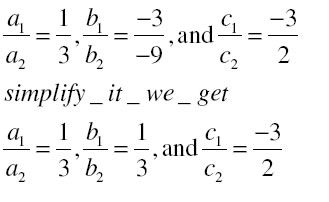HenceSo system has no solutions
(ii)
2x + y = 5
3x + 2y = 8

2x + y - 5 = 0
3x + 2y - 8 = 0

Compare withwe get
a1 = 2 , b1 = 1 , and c1 = -5
a2 = 3 , b2 = 2 and c2 = -8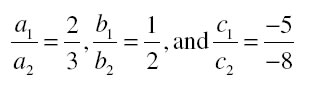HenceSo system has unique solutions
Use the formula of cross multiplication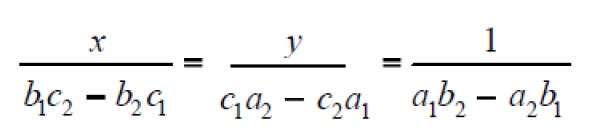Plug the values in this formula we get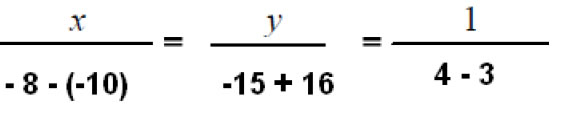Solve them we get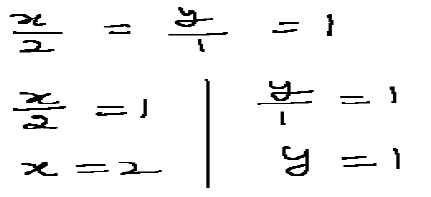Answer x = 2 and y = 1

(iii)
3x – 5y = 20
6x – 10 y = 40

3x – 5y - 20 = 0
6x – 10 y - 40 = 0
Compare withwe get
a1 = 3 , b1 = -5 , and c1 = -20
a2 = 6 , b2 = -10 and c2 = -40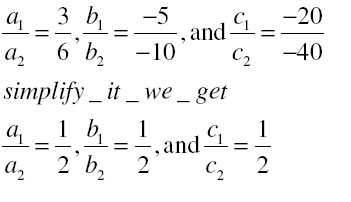HenceSo both lines are coincident and overlap with each other
So it will have infinity or many solutions

(iv)
X – 3y - 7 = 0
3x – 3y -15 = 0
Compare withwe get
a1 = 1 , b1 = -3 , and c1 = -7
a2 = 3 , b2 = -3 and c2 = -15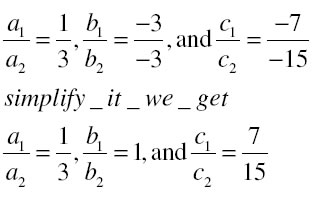HenceSo it will have unique solutions
Use the formula of cross multiplicationPlug the values in this formula we get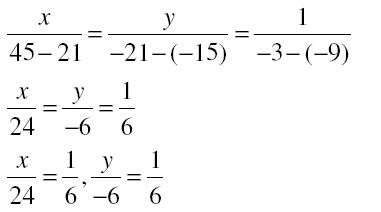X = 24/6 = 4
Y = -6/6 = - 1
So our answer is x = 4 and y = - 1

Question 2. (i) For which values of a and b does the following pair of linear equations have an infinite number of solutions?
2x +3y =7; (a – b) x +(a +b) y =3a +b –2

Solution: 2x +3y =7
Subtract 7 both side we get
2x + 3y - 7 = 0
(a – b) x +(a +b) y =3a +b –2
Subtract 3a + b -2 both side we get
(a – b) x +(a +b) y - (3a + b – 2) = 0

Compare with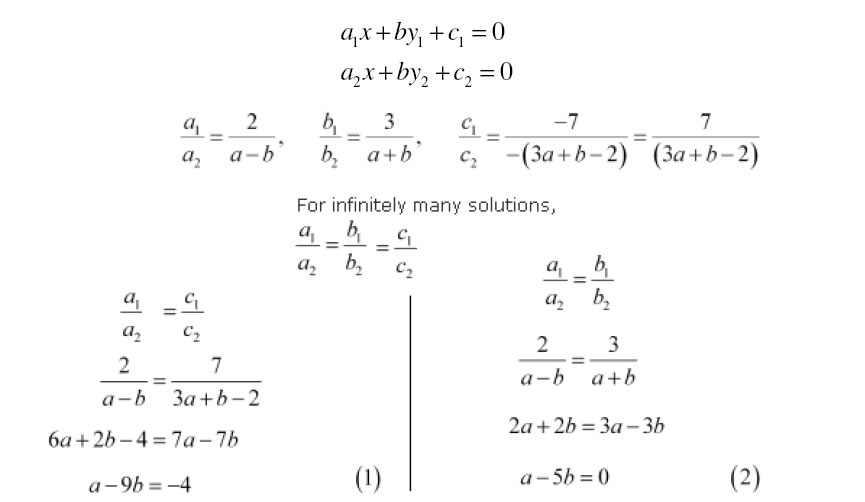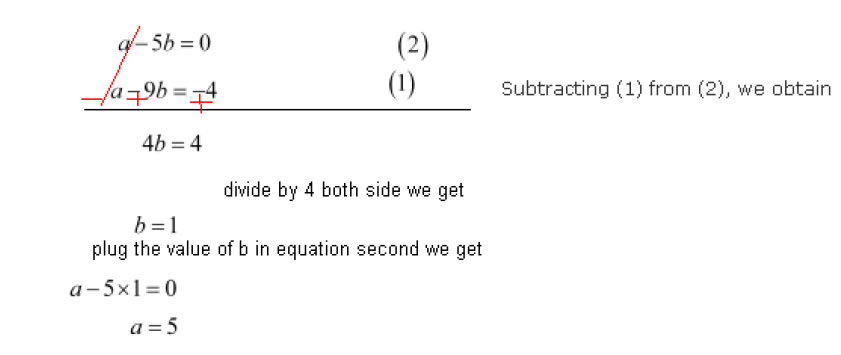Hence answer is a = 5 and b = 1

(ii) For which value of k will the following pair of linear equations have no solution?
3x +y = 1 ; (2k –1) x + (k –1) y = 2k + 1
3x +y = 1
Subtract 1 both side we get
3x + y – 1 = 0
(2k –1) x + (k –1) y = 2k + 1
Subtract 2k +1 both side we get
(2k –1) x + (k –1) y – (2k + 1)= 0
3x+y=1 and
(2k-1)x+(k-1)y=2k+1
They can be rewrite as:
3x + y - 1 = 0 and
(2k-1)x + (k-1)y - (2k+1) = 0
Compare withWe get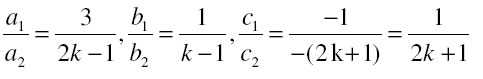And for no solutionTake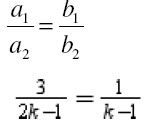Cross multiply we get
3k - 3 = 2k - 1
k = 3-1
k = 2
Hence Answer is k = 2

Question 3. Solve the following pair of linear equations by the substitution and cross-multiplication methods:
8x +5y =9 …(1)
3x +2y =4 …(2)

Solution: Substitution method :-
8x +5y =9
Subtract 8x both sides we get
5y = 9 – 8x
Divide by 5

Y = (9 – 8 x)/5 …(3)
Plug this value of y in equation second we get
3x +2y =4
3x + 2(9 – 8 x)/5) = 4
Multiply by 5 we get
15 x + 18 - 16 x = 20
-x = 2
X = - 2
Plug this value back in equation (3) we get
Y = (9- 8(-2))/5
Y = 25/5
Y = 5
Answer is x = -2 and y = 5
Cross multiplication method
8x +5y =9 …(1)
Subtract 9 both side we get
8x +5y – 9 = 0
3x +2y =4 …(2)
Subtract 4 both side we get
3x + 2y – 4 = 0
Compare withwe get
a1 = 8 , b1 = 5 , and c1 = -9
a2 = 3 , b2 = 2 and c2 = -4

Use the formula of cross multiplication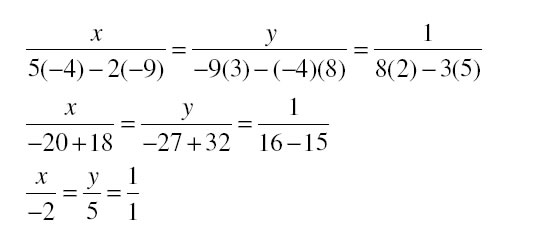We get x = -2 and y = 5

Question 4. Form the pair of linear equations in the following problems and find their solutions (if they exist) by any algebraic method :
(i) A part of monthly hostel charges is fixed and the remaining depends on the number of days one has taken food in the mess.When a student Atakes food for 20 days she has to pay Rs 1000 as hostel charges whereas a student B, who takes food for 26 days, paysRs 1180 as hostel charges. Find the fixed charges and the cost of food per day.

Solution: Let fixed charge = Rs x
Variable charge = Rs y per day
When a student Atakes food for 20 days she has to pay Rs 1000 as hostel charges
So we get
X + 20 y = 1000 …(1)
Subtract 20 y both side we get
X = 1000 – 20 y …(2)
who takes food for 26 days, paysRs 1180 as hostel charges
we get
x + 26 y = 1180
plug the value of x we ge t

1000 – 20 y + 26y = 1180
6y = 180
Y = 180/6 = 30

Plug the value of y in equation second we get
X = 1000 – 20 * 30
X = 1000 – 600
X = 400
Hence fixed charge x = Rs 400
And variable charge y = Rs 30

(ii) A fraction becomes 1/3 when 1 is subtracted from the numerator and it becomes 1/ 4 when 8 is added to its denominator. Find the fraction.
Let fraction is x/y
A fraction becomes 1/3 when 1 is subtracted from the numerator
So that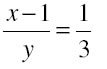Cross multiply we get
3x -3 = y …(1)
and it becomes 1/ 4 when 8 is added to its denominator
so that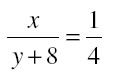4x = y + 8
Plug the value of y from equation first we get
4x = 3x – 3 + 8
X = 5
Plug this value back in equation first we get
3*5 – 3 = y
15 – 3 = y
12 = y
Hence fraction is x/y = 5/12

(iii) Yash scored 40marks in a test, getting 3marks for each right answer and losing1 mark for each wrong answer. Had 4marks been awarded for each correct answer and 2 marks been deducted for each incorrect answer, then Yash would have scored 50 marks. How many questions were there in the test?
Let correct question = x
And incorrect question = y
Yash scored 40 marks in a test, getting 3marks for each right answer and losing1 mark for each wrong answer.
3x - y = 40
Subtract 3x both sides we get
-Y = 40 – 3x
Change the sign we get
Y = 3x – 40 …(1)
Had 4 marks been awarded for each correct answer and 2 marks been deducted for each incorrect answer, then Yash would have scored 50 marks
We get
4x – 2y = 50
Plug the value of y we get
4x – 2(3x - 40) =50
4x – 6x + 80 = 50
-2x = - 30
X = 15
Plug this value back in equation first we get
Y = 3x – 40
Y = 3* 15- 40
Y = 45 – 40
Y = 5
So total question are x + y = 15 + 5 = 20

(iv) Places A and B are 100 km apart on a highway. One car starts from A and another from B at the same time. If the cars travel in the same direction at different speeds, they meet in 5 hours. If they travel towards each other, they meet in 1 hour. What are the speeds of the two cars?
Let speed of faster car = x Km/ h
Speed of slower car = y km /h
Relative speed when both car travels in same direction = x - y
If the cars travel in the same direction at different speeds, they meet in 5 hours
Speed = distance/ time
Total distance = 100 km
Speed x – y = 100/5
X – y = 20
Add y both side we get
X = 20 + y …(1)
Relative speed when both car travels in opposite direction = x + y
If they travel towards each other, they meet in 1 hour.
Speed = distance/ time
Total distance = 100 km
Speed x + y = 100/1
Plug the value of x form equation first we get
20 + y + y = 100
2y = 80
Y = 40
Plug the value of y in equation first we get
X = 20 + 40
X = 60
Hence speeds are 40 Km /h and 60 Km /h

(v) The area of a rectangle gets reduced by 9 square units, if its length is reduced by 5 units andbreadth is increased by 3 units. If we increase the length by 3units and the breadth by 2 units, the area increases by 67 square units. Find the dimensions of the rectangle.
Let length of rectangle = x units
And width of rectangle = y units
Area of rectangle = length * width = x*y
The area of a rectangle gets reduced by 9 square units, if its length is reduced by 5 units and breadth is increased by 3 units.
So
Decrease the length by 5 unit so new length = x - 5
Increase the width by 3 unit so new width = y + 3
New area is reduced by 9 units
So new area = xy – 9
Plug the value in formula length * width = area we get
(x - 5)(y + 3) = xy - 9
Xy + 3x – 5y – 15 = xy – 9
Subtract xy both side we get
3x - 5y = 6 …(1)
If we increase the length by 3units and the breadth by 2 units, the area increases by 67 square units.
Increase the length by 3 unit so new length = x +3
Increase the width by 2 unit so new width = y + 2
New area is increased by 67 units
So new area = xy + 67
Plug the value in formula length * width = area we get
(x +3)(y + 2) = xy + 67
Xy + 2x + 3y + 6 = xy + 67
Subtract xy both side we get
2x + 3y = 61 …(2)*3
3x - 5y = 6 …(1)*2
Cross multiply the coefficient of x we get
6x + 9 y = 183
6x -10y =12
Subtract now we get
19 y = 171
Y = 171/19 = 9
Plug this value of y in equation first we get
2x + 3* 9 = 61
2x = 61 – 27
2x = 34
X = 34/2 = 17
So length is 17 units and width is 9 units

### NCERT Books Free Pdf Download for Class 5, 6, 7, 8, 9, 10 , 11, 12 Hindi and English Medium

 Mathematics Biology Psychology Chemistry English Economics Sociology Hindi Business Studies Geography Science Political Science Statistics Physics Accountancy